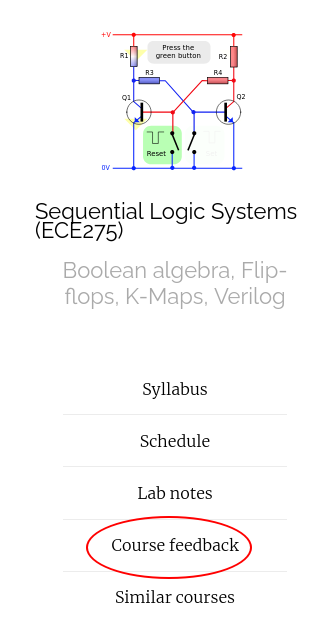## Announcemnts

• Midterm 2 postponed on Nov 15th.
• HW 8 is posted is due next Wednesday (Nov 10th) at 9 AM before class.

## Ex2: Parity Checker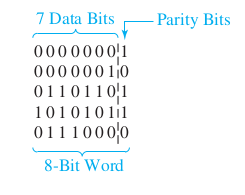## Ex2: Parity Checker using T flip-flops## Ex2: Parity Checker using J-K flip-flops## Ex2: Parity Checker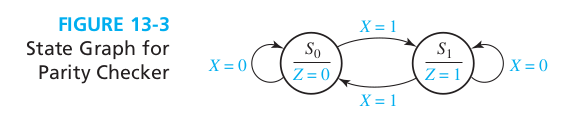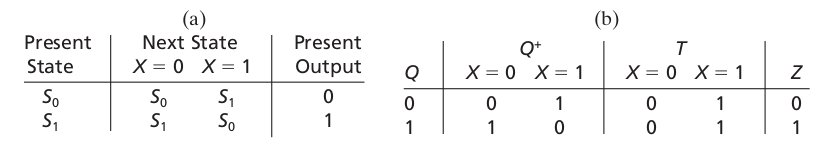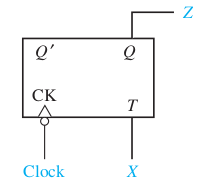## State reduction: Ex1

A sequential circuit has one input (X) and one output (Z). The circuit examines groups of four consecutive inputs and produces an output $$Z = 1$$ if the input sequence 0101 or 1001 occurs. The circuit resets after every four inputs. Find the Mealy state graph.

## State reduction: Ex1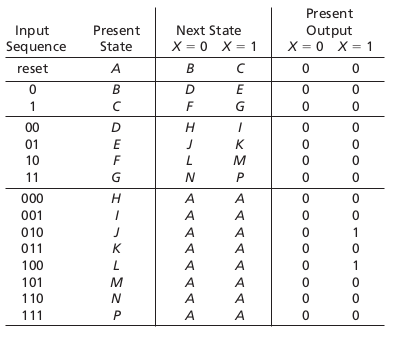## State reduction: Ex1## State reduction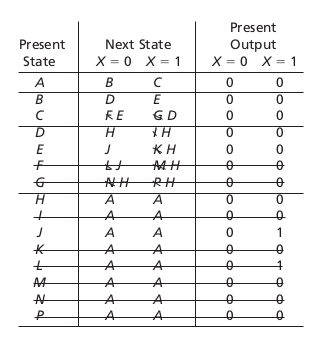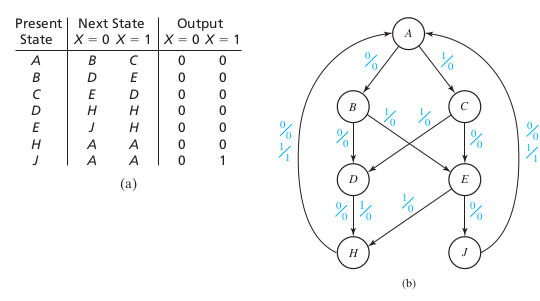## Equivalent states: Defn

• Let $$N_1$$ and $$N_2$$ be sequential circuits (not necessarily different).
• Let $$\underline{X}$$ represent a sequence of inputs of arbitrary length.
• Let $$\underline{Z}_1 = \lambda_1(p, \underline{X} )$$ the sequence of outputs corresponding to $$\underline{X}$$ starting at state state $$p$$ for circuit $$N_1$$. Let $$\underline{Z}_2 = \lambda_2(p, \underline{X} )$$ denote the same for circuit $$N_2$$.
• Then state $$p$$ in $$N_1$$ is equivalent to state $$q$$ in $$N_2$$ iff $$\lambda_1(p, \underline{X} ) = \lambda_2 (q, \underline{X})$$ for every possible input sequence.
• Symbol: $$p \equiv q$$

## Equivalent states: Thm

Two states $$p$$ and $$q$$ of a sequential circuit are equivalent iff for every single input $$X$$, the outputs $$\lambda(p, X)$$ are the same and the next states $$p_{t+1} = \phi(p, X)$$ are equivalent, that is,
• $$\lambda(p, X) = \lambda(q, X)$$
• and $$\phi(p, X) = \phi(q, X)$$.

## Implication tables: Ex2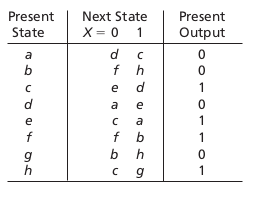## Implication tables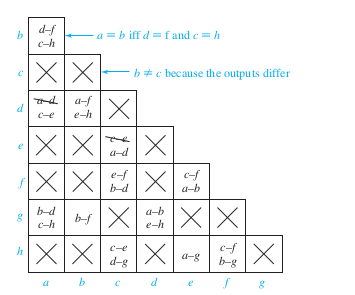## Implication tables algorithm

1. Construct a chart which contains a square for each pair of states.
2. Compare each pair of rows in the state table. If the outputs associated with states i and j are different, place an X in square i-j to indicate that $$i\equiv j$$.
3. If the outputs are the same, place the implied pairs in square i-j. (If the next states of i and j are m and n for some input x, then m-n is an implied pair.)
4. If the outputs and next states are the same (or if i-j only implies itself), place a check (√) in square i-j to indicate that $$i \equiv j$$
5. Go through the table square-by-square. If square i-j contains the implied pair m-n, and square m-n contains an X, then i [ j, and an X should be placed in square $$i \equiv j$$.
6. If any X’s were added in step 3, repeat step 3 until no more X’s are added.
7. For each square i-j which does not contain an X, $$i \equiv j$$.

## Equivalent State assignments

• Swapping flip-flops are equivalent.
• Inverting flip-flop are equivalent.

## Equivalent State assignments: Ex1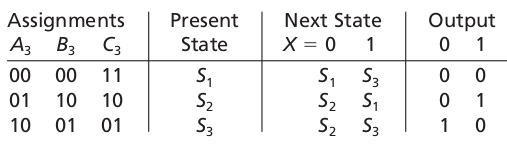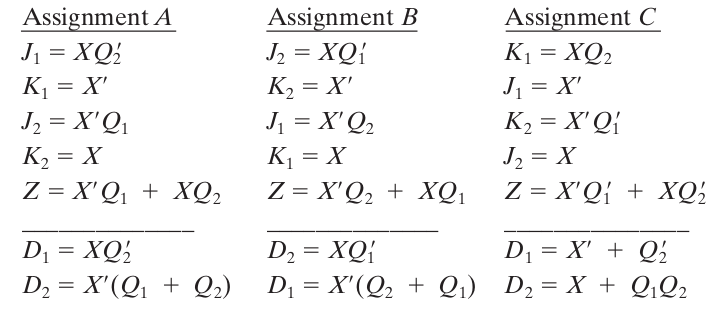## Distict state assignments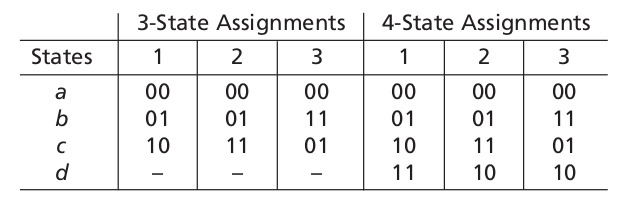## Distict state assignments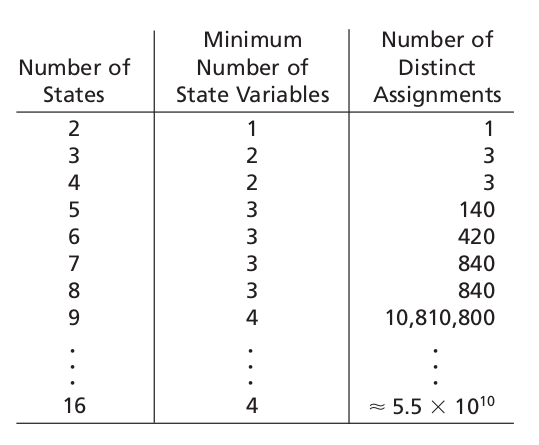## Guidelines for state assignment

• (Group in-neigbours) States which have the same next state for a given input should be given adjacent assignments.
• (Group out-neighbors) States which are the next states of the same state should be given adjacent assignments.
• States which have the same output for a given input should be given adjacent assignments.

## State assignment: Ex1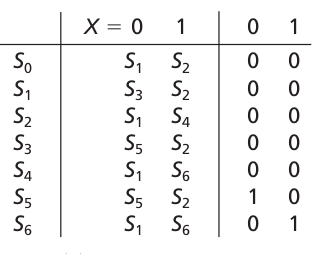## Thanks, Questions, Feedback?

https://vikasdhiman.info/ECE275-Sequential-Logic/